# Construction and Definition of Numbers

The concept of numbers is way more complicated than it sounds. In this article, we will provide an introduction to how they are mathematically constructed from the most fundamental level in set theory. This should give you an insight into what they truly mean and are. Beware that, as a result, this article is relatively technical.

## Natural Numbers

Natural numbers appear to us as the simplest numbers. They seem to be the fundamental blocks of mathematics. But they are not. Nearly all mathematical theories are rather based on set theory. In these theories, all mathematical objects are sets. The way they interact is then defined by the axioms of the theory. In this context, natural numbers exist only if these axioms allow the construction of sets which perfectly match what we would expect from natural numbers.

Well, it shouldn’t be too hard to find such axioms…

Well, in fact, it’s a pretty tough task to find such axioms which don’t imply any contradiction… which is essential! In 1901, Bertrand Russell proved the necessity of doing this carefully, as he showed that the axioms of the time, now known as naive set theory, led to the Russell’s paradox. This led to the foundational crisis of mathematics: During the following decades, mathematicians were lost, as no appropriate set of axioms were found.

What about now? Have they found the appropriate set of axioms?

Well, they found a set of axioms that seems appropriate, called the Zermelo–Fraenkel set theory with the axiom of choice, or ZFC. ZFC comes from Zermelo’s first axioms introduced in 1908 and was then slowly improved until the 1920s.

What do you mean by “seems appropriate”? We don’t even know???

Hehe… Let’s not get too much into this because it would take a while! You can learn more on the axiomatization of mathematics in my article on self-reference. Let’s now just stick with ZFC. Now, the question is: How can we define sets which correspond to natural numbers? And we need a preliminary result…

What’s the preliminary result?

The existence of the empty set, noted $\emptyset$. Shortly put, the existence of such set comes from three axioms. First, we need to assume that there exists at least one set. This is natural, as otherwise, the theory would be about nothing. Second, we need to use the axiom schema of specification, which is a handy way of constructing the subset of a set which satisfy some property. Third and finally, we need the axiom of extensionality, which defines equality. The empty set can then be defined as the set of all elements of the existing one which are not equal to themselves.

OK, the empty set exists… What now?

Next, we can show that there exists another set constructed based on the empty set. To do this, we need the axiom of power set. It says that, for any set, the set of all its subsets exists. This set of all subsets is called the power set. By combining this axiom with the axiom schema of specification, we can also prove that, for any set, there exists a set which contains this set only. Formally, if $x$ is a set, this says that the set $\{x\}$ which contains $x$ only exists. This set is defined as the set of all subsets of $x$ which are equal to $x$, that is, formally, $\{x\}$=$\{y∈P(x)$|$y=x\}$, where $P(x)$ is the power set. Don’t worry about the formulas if you don’t understand them, just think of them as a decoration for this article!

OK…

Now, we can repeat the construction of the set $\{x\}$ with every set $x$ we have constructed, starting with the empty set. If we now say that the empty set corresponds to 0, that the set of the empty set is 1, that the set of the set of the empty set is 2, and so on… we can then match every set we have constructed with a natural number! Natural numbers have been constructed! All basic relations like order relation, addition and multiplication between natural numbers can then be defined based on this construction!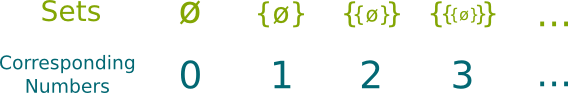There are plenty of other ways to construct natural numbers. The most common was introduced by John von Neumann, and others are listed here. But these other constructions require the axiom of union. Also, the Bourbaki group proposed another construction based on cardinals, but I don’t know the rigorous construction. Finally, recently, a very different approach was given by type theory.
Great! So we’re done with the set of natural numbers, right?

No! Although each natural number has been constructed. They cannot be grouped in a set of natural numbers! And that’s actually quite an issue, as so far, all sets we can construct are necessarily finite. To construct the set of natural numbers, we need a new axiom, called the axiom of infinity. And with this axiom, we can collect all natural numbers in a set which perfectly matches the expected properties of the set of natural numbers.

Any such set is said to be isomorphic to the set of natural numbers. This means that, once the set of natural numbers is constructed, it can be thought independently from its construction and should rather be thought in terms of its properties. The set of natural numbers is thus commonly denoted $\mathbb N$, and it’s used regardless to the way we have constructed it.

Note that in computer science, because memory space is finite, the axiom of infinity is not satisfied! This means that ZFC is wrong to describe what’s inside the computer. Typically, in a computer, natural numbers exist up to the capacity of the memory, as well as rational numbers whose precision is also limited by memory, but real numbers don’t! But approximations of what’s happening inside the computer with ZFC are good though, and they can be more practical to deal with.
Did it have to be so complicated?

For things to be perfectly well-defined from the basics of logics, yes! But as I’ve said this beautiful definition only came in the 20th century. For a long long time, numbers were poorly defined, which led to fuzzy reasonings. In particular, German mathematician Georg Cantor showed that mathematics were way more complicated than what people thought, with counter-intuitive sets like Cantor’s set and the construction of different infinities, as displayed in the following video by Ted Ed.

In the late 1800s, because of Cantor’s work, it became more and more important to better define mathematics. In fact, mathematics were so poorly defined that it implied paradoxes were found. Even today, infinity has some disturbing consequences, such as the result $1+2+4+8+16$+$…=-1$! Find out more with my article on the surprising flavor of infinite series. For this quirkiness to still make sense, it’s of the greatest importance that the setting in which infinity and numbers are defined shall be very rigorous.

And Cantor provided such a rigorous construction?

No! The first major breakthrough was made by Italian mathematician Giuseppe Peano, as he defined Peano’s axioms in 1889. These are the simplest axioms required to define the set of natural numbers. Instead of having them as axioms, ZFC makes them consequences of its more fundamental axioms. This can be compared to the way DNA theory makes the evolution of species an aftermath of its postulates.

An important consequence of Peano’s axioms is the proof by induction, which is extremely powerful to solve many problems.

## Integer Numbers

Let’s go further with integer numbers! As you probably know, the set of natural numbers can be completed with negative numbers to form the set of integer numbers. But do these integer numbers actually exist in ZFC theory?

I hope so!

If they didn’t, then ZFC wouldn’t be good… So the answer is yes, they do exist in ZFC. Let’s see how they can be constructed! First, we need to use the axiom of pairing. This axiom says that we can define the cartesian product of two sets, which is the set of pairs whose first element belongs to the first set, and whose second element belongs to the second set. The product set of two sets $A$ and $B$ is denoted $A \times B$. Let’s apply this to the set of natural numbers, to define the set of pairs of natural numbers.

OK, so the set of pairs of natural numbers exists… I don’t see how this is related to integer numbers.

We’ll get there. But before getting there, we need to introduce the concept of binary relation over a set. This binary relation compares any two elements of the set. It says if the first element is in relation with the second. An example is the order relation for natural numbers, which says whether the first element is greater than the second. Another example is the equality relation for natural numbers, which says whether the first element is equal to the second.

But do binary relations exist in ZFC?

Yes! In set theory, a binary relation is defined as a subset of the cartesian product of the set with itself. A pair of elements of a set are related by a binary relation if and only if the pair belongs to the representation of the binary relation as a subset. This is displayed in the following figure: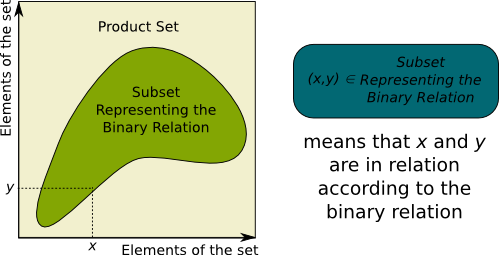Try to draw the subset representation of equality relation and order relation for natural numbers. You should obtain the bottom-left to top-right diagonal in the first case, and the upper top-left triangle in the second.

Other mathematical objects are defined similarly to binary relations. For instance, a function of a set $A$ to a set $B$ is a subset of the cartesian product $A \times B$ such that for every $a \in A$, there exists a unique related $b \in B$. Once again, in set theory, every object is a set!
I don’t see what binary relations have to do with our constructions of integer numbers…

We will now define a binary relation between the pairs of natural numbers. We’ll say that two pairs of natural numbers $(a,b)$ and $(c,d)$ are related if $a+d$=$b+c$. For instance, $(4,1)$ and $(9,6)$ are related because $4+6$=$10=1+9$.

OK…

A major property is that the binary relation is an equivalence relation. This means that it satisfies the three following properties:

• reflexivity: All pairs are in relation with themselves.
• symmetry: If a first pair is in relation with a second, then the second is in relation with the first too.
• transitivity: If a first pair is in relation with a second, and if a second is in relation with a third, then the first is in relation with the third.

I’ll let you prove that these three properties are indeed satisfied. Because this binary relation is an equivalence relation, we can simply say that two elements in relation are equivalent. Now, what’s great with this property is that it implies that we can separate the set of all pairs into subsets such that elements of a subset are all equivalent. Each of these subsets is called an equivalence class, and the set of all equivalence classes is called the quotient space.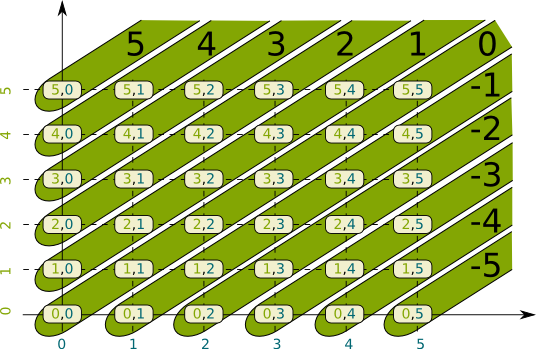The equivalence classes are the grouping made on the figure. You should check by yourself that they are indeed equivalence classes, that is, all elements of each grouping are equivalent.

The equivalence classes correspond to integer numbers. The set of the equivalence classes, called the quotient space, is the set of integer numbers, commonly denoted $\mathbb Z$. It’s interesting to note that, when the first element of the pair is greater than the second, then the equivalence class perfectly matches natural number equal to the difference between the two numbers. Based on this construction, order relation, addition and multiplication for integer numbers can then be defined.

In 2013, a homotopy type theorist consortium has introduced a new approach to mathematics, which yields a more computable and intuitive definition of integers.

On a more technical level, it’s also interesting to notice that negative numbers help make the set of natural numbers an abelian additive group! This means that equations like $x+3=0$ now have solutions, as well as any equations where 3 is replaced by any other integer number. In abstract algebra, this makes the set of integer numbers a commutative ordered ring.

## Rational Numbers

The common extension of rings is to try to make them fields. This would enable equations like $4x+3=0$ to have solutions, where numbers 4 and 3 can be replaced by any integer numbers (except that 4 cannot be replaced by 0). To do so, we need to introduced rational numbers, which are sort of ratios of two integer numbers… although this ratio is not uniquely defined by the choice of the two integers!

This sounds like we should use equivalence classes…

Yes! First, we need to consider the set of pairs of an integer number and a positive natural number (different from 0). We then consider the equivalence relation over this set of pairs, for which $(a,b)$ and $(c,d)$ are equivalent if $ad=bc$. After showing that this is indeed an equivalence relation, we define the set of rational numbers as the quotient space. The equivalence classes are structured as follows: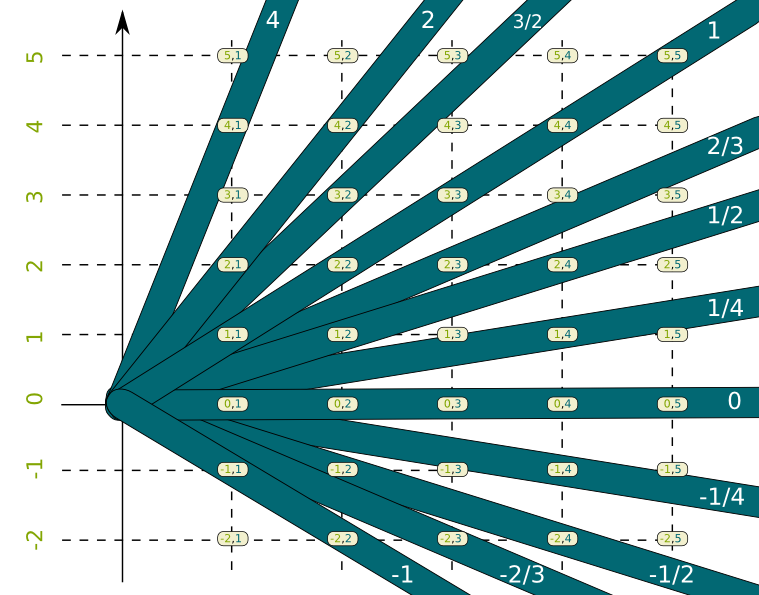It might look like these equivalence classes overlap, but they don’t. The figure displays them overlapping at the point (0,0), but this point is not in the set of pairs we considered so it doesn’t count. I have just extended them to show the structure of the equivalence classes. It’s important to notice that the second element of pairs is never zero. That’s good because you should NEVER DIVIDE BY ZERO.

You can then extend the definitions of order relation, addition and multiplication to these equivalence classes. This defines the set of rational numbers, commonly denoted $\mathbb Q$. This set is now a field, which means that in addition to properties of integers we mentioned, every number can now be a multiplied by a rational number such that the product is equal to 1. As a result, all equations $ax+b=0$ have solutions when $a$ and $b$ are rationals (and $a$ is non-zero!).

## Real Numbers

Pythagorean believed that all numbers were rational. It was even part of their philosophy. So much that some historians believe that one of them, Hippasus, was drowned when he proved the irrationality of the square root of 2! It is now known that the square root of 2 is not the only number which is not rational. Other major numbers of mathematics are not, such as $\pi$ and $e$.

But they do exist… I mean, there has to be a way to construct them!

And there is. The construction I was introduced to is based on the idea of the Greek scholar Archimedes. He tried to find $\pi$ by improving its approximations with rational numbers, by inserting polygons inside and outside a circle. Archimedes’ idea is described in the following video by Numberphile:

Formally, saying that real numbers are numbers which can be approached by rational numbers corresponds to using Cauchy sequences of rational numbers. They are sequences which stabilize enough, and should therefore converge. But their limit is not rational. But what we can do is consider the set of all Cauchy sequences. On this set, we define the equivalence relation which is true if the difference between two sequences converges to 0. The set of real numbers is then defined as the quotient space of Cauchy sequences.

I’m lost! You went to fast!!!

I know. The problem is that defining Cauchy sequences is a little bit abstract. Instead, I’m going to present you another construction of real numbers! Consider the set of all subsets of rational numbers which are upper bounded.

What’s an upper bounded subset?

It’s a set such that there exists a rational number, called upper bound, which is higher than all elements of the subset. Basically, upper bounded subsets don’t have elements which go to infinity. Now, you could order the subsets of rational numbers by saying that one subset is greater than the other if it has an element which is an upper bound for the other. But it’s possible that none of two subsets is greater than the other. This would mean that all elements of the former is smaller than an element of the latter, and all elements of the latter is smaller than an element of the former. Such two subsets can be called equivalent. This is displayed in the following figure.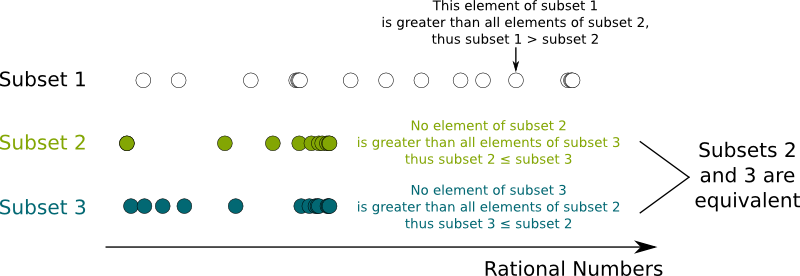And I guess that the set of real numbers is the quotient space once again…

Yes! Real numbers correspond to equivalence classes of upper-bounded subsets of rational numbers. The order relation we have described perfectly match the order relation between real numbers. Addition can easily be defined based on this idea. But you will have to be a bit more careful with multiplication, especially when dealing with negative numbers!

Note that the construction of real numbers requires to match them with infinite subsets of rational numbers. This is why the axiom of infinity is needed to construct real numbers. It’s also the reason why the cardinality of the set of real numbers is greater than the cardinality of rational numbers.

The set of real numbers denoted $\mathbb R$ is extremely important in abstract algebra, analysis and topology, as well as most areas of mathematics, because just like the set of rationals, it’s a commutative ordered field. But what’s more, it is complete, which means that all Cauchy sequences converge to a real number. This is crucial in analysis like differential calculus and topology.

## Complex Numbers

Historically, the need to extend the set of real numbers appeared when mathematicians wanted to be able to solve all equations. In particular, they sought for a solution to the equation $x^2=-1$. But as you’ve probably guessed, simply assuming that an imaginary number $i$ is the solution of this equation is not satisfying in a well-defined set theory. We need to construct a set which contains real numbers as well as an element which will have the property of being solution of this equation!

So how can the imaginary number i be constructed?

Once again, there are plenty of approaches to do this. Just like for Cauchy sequences, I’ll now very briefly present the most elegant construction I know (and you’ll need to dig in a little bit of abstract algebra to understand it!). It consists in constructing the set of polynomials with real coefficients. Then we define addition and multiplication for polynomials, and show that they make this set a commutative ring. We then notice that the fact that $x^2+1=0$ implies that the polynomial $X^2+1$ is a prime polynomial, in the sense that there does not exist a pair of non-trivial polynomials different 1 whose product is $X^2+1$. This implies that the set of multiples of $X^2+1$ is a maximal ideal of the ring of real polynomials. We can then define the congruence relation between polynomials: Two real polynomials are congruent if their difference is in the maximal ideal. This congruence relation is an equivalence relation. The quotient space of the ring of real polynomials by this congruence relation can then be proved to be a commutative field, which perfectly matches what we expect from complex numbers.

OK… Can you present a simpler approach?

Sure. The set of complex numbers is basically the product of the set of real numbers by itself. The tricky thing regards multiplication for these pairs of real numbers. This is done by forcing that $(a,0) \times (b,0)$=$(ab,0)$, $(a,0) \times (0,b)$=$(0,ab)$ and $(0,a) \times (0,b)$=$(-ab,0)$. This construction works perfectly, but it looks more arbitrary than the previous one…

But I thought that complex numbers would be an extension of real numbers… There’s no real numbers among pairs of real numbers!

Yes, you’re right. We do so by associating every pair $(a,0)$ with the real number $a$. This way, every real number has exactly one equivalent in terms of pairs of numbers. This technic is called a canonical injection. And you may now notice that $(0,1) \times (0,1)$=$(-1 \times 1,0)$=$(-1,0)$=$-1$!!! This means that the complex number $(0,1)$ is a square root of $-1$!

So (0,1) is equal to the imaginary number i?

Hum… You may notice that the square of $(0,-1)$ is also equal to $-1$… So you can pick any of the two complex numbers and call it $i$. As you’ve seen $i$ cannot (and should not) be called the square root of $-1$, because there are two of them! In particular, that’s why I get irritated when I see people write $i = \sqrt{-1}$. Arg… So ugly!

Still, I’m not used to write complex numbers as pairs of real numbers… I’m more used to writing them as a+ib!

Indeed you can! Let’s say that $i$ is $(0,1)$. Then $(a,b)$=$(a,0)$+$(0,b)$=$(a,0)$+$(0,1) \times (b,0)$=$a+ib$. This shows that all complex numbers can be rewritten $a+ib$, as it’s done in textbooks. Happy?

Very! So the point of these complex numbers is to solve all equations?

Humm… All equations don’t have a complex solution. But all polynomial equations now do! That’s what the set of complex numbers has been created for. We say that it’s the algebraic closure of the field of real numbers. But the main use of complex numbers is rather to describe oscillations, especially in physics, through the marvels of Fourier analysis. They are also very useful to solve differential equations and find eigenvalues in quantum mechanics. In fact, in quantum mechanics, complex numbers are essential to define the Hilbert space in which particles live. Find out more with my article on the dynamics of the wave function.

For a more insightful and more complete description of complex numbers, check my article on the topic. There’s also this article on Euler’s identity.

## Let’s Conclude

I bet you didn’t expect numbers to be that complicated! What may be surprising is to notice that the difficulty of the construction of a number is not directly related to the difficulty of conceiving it. In particular, in terms of topology and calculus, real numbers are much much simpler than rational numbers, although they are awfully more complicated to construct. On the opposite, we have trouble to conceive complex numbers, even though their construction and manipulation are relatively simple.

Now, my geeky side can’t resist writing down the actual formulas which define numbers. There are several symbols I haven’t explained, so it’s normal if you don’t understand them! But if you go to the wikipedia pages of the concepts of this article, then you can learn to read the formulas! And if you do understand them, then this means that (almost) nothing can stop you from understanding anything and becoming a mathematician!Note that, to construct numbers, I didn’t think use the following axioms: axiom of regularity, axiom of union, axiom schema of replacement and axiom of choice.

Historically, numbers have been defined to solve equations. However, their constructions follow algebraic ideas which aim at adding more nice properties to the set of numbers. And there are plenty of variants of these ideas, which lead to the construction of many more numbers with totally different properties, such as the set of integers modulo $p$ denoted $\mathbb Z/p\mathbb Z$, the set of p-adic numbers $\mathbb Q_p$ or the set of hyperreal numbers $^*\mathbb R$. Also, interestingly, hardly none of real numbers are in fact definable mathematically! Find out more with the sequel of this article, as I explore the constructibility of numbers.

## More on Science4All

The Magic of AlgebraBy Lê Nguyên Hoang | Updated:2016-02 | Views: 3796
The power of algebra lies in abstraction, and abstraction is basically forgetting. By retracing the History of algebra from its roots to more recent advancements, this article unveils the numerous breakthrough in our understanding of the world, by abusing of the power of forgetting.

Self-Reference, Math Foundations and Gödel's IncompletenessBy Lê Nguyên Hoang | Updated:2016-02 | Views: 6399
Although highly appreciated by artists, self-reference has been a nightmare for mathematicians. It took one of the greatest, Kurt Gödel, to provide a better understanding of it. This article deals with paradoxes, recursion, fractals and Gödel's incompleteness theorems.

Numbers and ConstructibilityBy Lê Nguyên Hoang | Updated:2016-02 | Views: 9099
Last summer, I got to discover Morellet's artwork on inclined grids. Amazingly, this artwork is a display of the irrationality of $\sqrt{2}$! It's also a strong argument for the existence of this number. In this article, after discussing that, I take readers further by discussing what numbers can be constructed geometrically, algebraically, analytically or set theoretically using the power of mathematics!

Proof by Mathematical InductionBy Lê Nguyên Hoang | Updated:2015-12 | Views: 6562
This article explores the potency of proofs by induction with 4 different stunning puzzles, from a lock puzzle and a lion issue, to the monk problem and the pencil conundrum!

Type Theory: A Modern Computable Paradigm for MathBy Lê Nguyên Hoang | Updated:2016-01 | Views: 12657
In 2013, three dozens of today's brightest minds have just laid out new foundation of mathematics after a year of collective effort. This new paradigm better fits both informal and computationally-checkable mathematics. There is little doubt that it will fundamentally change our perspective on rigorous knowledge, and it could be that, in a few decades, the book they published turns out to be the bedrock of all mathematics, and, by extension, all human knowledge! Have a primer of this upcoming revolution, with this article on type theory, the theory that the book builds upon!

The Limitless Vertigo of Cantor's InfiniteBy Lê Nguyên Hoang | Updated:2015-12 | Views: 3899
No one believed him. Not even fellow mathematicians. They thought he was wrong. They thought he was crazy. Even he ended up doubting himself and went crazy. And yet, he had mathematically proved it all. Georg Cantor had figured out how to manipulate the infinite. Even more remarkable, he showed that there were actually several infinities; and some are bigger than others!

Imaginary and Complex NumbersBy Lê Nguyên Hoang | Updated:2016-02 | Views: 6776
My first reaction to imaginary numbers was... What the hell is that? Even now, I have trouble getting my head around these mathematical objects. Fortunately, I have a secret weapon: Geometry! This article proposes constructing complex numbers with a very geometrical and intuitive approach, which is probably very different from what you've learned (or will learn).

The Most Beautiful Equation of Math: Euler's IdentityBy Lê Nguyên Hoang | Updated:2016-01 | Views: 92459
In 1988, Euler's identity was elected most beautiful theorem of mathematics. It has been widely taught worldwide. But have you ever stopped to really sense the meaning of this incredible formula? This article does.

## More Elsewhere

1.scottmckinney says:
2.juanpa says: